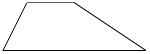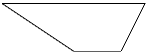Study Guide

# Basic Geometry - Congruent Figures

## Congruent Figures

Congruent figures have the same shape and size, they're totally equal. Same-same. All of the sides on one figure are equal to all of the corresponding side on the other figure, and all of the angles of one figure are equal to all of the corresponding angles on the other figure. Samesies. The sides are congruent and the angles are congruent. Even if the shapes are rotated so they face different directions, the shapes are congruent if their sides and angles are the same. The shapes below are congruent, which is shown by the symbol ≅.